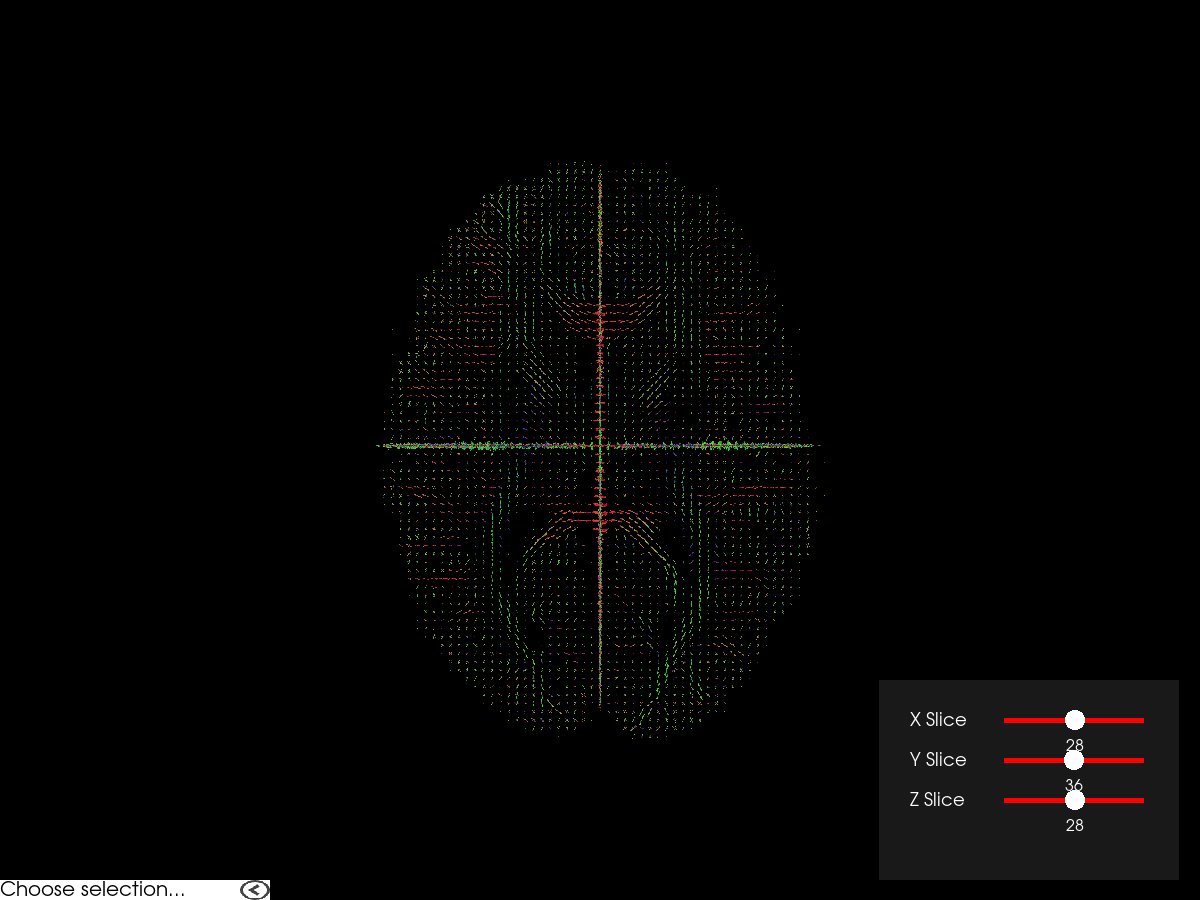# Brain Fiber ODF Visualisation¶

This example demonstrate how to create a simple viewer for fiber orientation distribution functions (ODF) using fury’s odf_slicer.

```# First, we import some useful modules and methods.
import numpy as np
import nibabel as nib
from fury import actor, window, ui
from fury.data import read_viz_dmri, fetch_viz_dmri, fetch_viz_icons
from fury.utils import fix_winding_order
from dipy.reconst.shm import sh_to_sf_matrix
from dipy.data import get_sphere
```

Here, we fetch and load the fiber ODF volume to display. The ODF are expressed as spherical harmonics (SH) coefficients in a 3D grid.

```fetch_viz_dmri()
fetch_viz_icons()

sh = fodf_img.get_fdata()
affine = fodf_img.affine
grid_shape = sh.shape[:-1]
```

Out:

```Dataset is already in place. If you want to fetch it again please first remove the folder /Users/koudoro/.fury/dmri
Data size is approximately 12KB
Dataset is already in place. If you want to fetch it again please first remove the folder /Users/koudoro/.fury/icons
```

We then define a low resolution sphere used to visualize SH coefficients as spherical functions (SF) as well as a matrix B_low to project SH onto the sphere.

```sphere_low = get_sphere('repulsion100')
B_low = sh_to_sf_matrix(sphere_low, 8, return_inv=False)
```

Now, we create a slicer for each orientation to display a slice in the middle of the volume and we add them to a scene.

```# Change these values to test various parameters combinations.
scale = 0.5
norm = False
colormap = None
opacity = 1.0
global_cm = False

# ODF slicer for axial slice
odf_actor_z = actor.odf_slicer(sh, affine=affine, sphere=sphere_low,
scale=scale, norm=norm,
colormap=colormap, global_cm=global_cm,
B_matrix=B_low)

# ODF slicer for coronal slice
odf_actor_y = actor.odf_slicer(sh, affine=affine, sphere=sphere_low,
scale=scale, norm=norm,
colormap=colormap, global_cm=global_cm,
B_matrix=B_low)
odf_actor_y.display_extent(0, grid_shape - 1, grid_shape//2,
grid_shape//2, 0, grid_shape - 1)

# ODF slicer for sagittal slice
odf_actor_x = actor.odf_slicer(sh, affine=affine, sphere=sphere_low,
scale=scale, norm=norm,
colormap=colormap, global_cm=global_cm,
B_matrix=B_low)
odf_actor_x.display_extent(grid_shape//2, grid_shape//2, 0,
grid_shape - 1, 0, grid_shape - 1)

scene = window.Scene()

show_m = window.ShowManager(scene, reset_camera=True, size=(1200, 900))
show_m.initialize()
```

Now that we have a ShowManager containing our slicer, we can go on and configure our UI for changing the slices to visualize.

```line_slider_z = ui.LineSlider2D(min_value=0,
max_value=grid_shape - 1,
initial_value=grid_shape / 2,
text_template="{value:.0f}",
length=140)

line_slider_y = ui.LineSlider2D(min_value=0,
max_value=grid_shape - 1,
initial_value=grid_shape / 2,
text_template="{value:.0f}",
length=140)

line_slider_x = ui.LineSlider2D(min_value=0,
max_value=grid_shape - 1,
initial_value=grid_shape / 2,
text_template="{value:.0f}",
length=140)
```

We also define a high resolution sphere to demonstrate the capability to dynamically change the sphere used for SH to SF projection.

```sphere_high = get_sphere('symmetric362')

# We fix the order of the faces' three vertices to a clockwise winding. This
# ensures all faces have a normal going away from the center of the sphere.
sphere_high.faces = fix_winding_order(sphere_high.vertices,
sphere_high.faces, True)
B_high = sh_to_sf_matrix(sphere_high, 8, return_inv=False)
```

We add a combobox for choosing the sphere resolution during execution.

```sphere_dict = {'Low resolution': (sphere_low, B_low),
'High resolution': (sphere_high, B_high)}
combobox = ui.ComboBox2D(items=list(sphere_dict))
```

Here we will write callbacks for the sliders and combo box and register them.

```def change_slice_z(slider):
i = int(np.round(slider.value))
odf_actor_z.slice_along_axis(i)

def change_slice_y(slider):
i = int(np.round(slider.value))
odf_actor_y.slice_along_axis(i, 'yaxis')

def change_slice_x(slider):
i = int(np.round(slider.value))
odf_actor_x.slice_along_axis(i, 'xaxis')

def change_sphere(combobox):
sphere, B = sphere_dict[combobox.selected_text]
odf_actor_x.update_sphere(sphere.vertices, sphere.faces, B)
odf_actor_y.update_sphere(sphere.vertices, sphere.faces, B)
odf_actor_z.update_sphere(sphere.vertices, sphere.faces, B)

line_slider_z.on_change = change_slice_z
line_slider_y.on_change = change_slice_y
line_slider_x.on_change = change_slice_x
combobox.on_change = change_sphere
```

We then add labels for the sliders and position them inside a panel.

```def build_label(text):
label = ui.TextBlock2D()
label.message = text
label.font_size = 18
label.font_family = 'Arial'
label.justification = 'left'
label.bold = False
label.italic = False
label.background_color = (0, 0, 0)
label.color = (1, 1, 1)

return label

line_slider_label_z = build_label(text="Z Slice")
line_slider_label_y = build_label(text="Y Slice")
line_slider_label_x = build_label(text="X Slice")

panel = ui.Panel2D(size=(300, 200),
color=(1, 1, 1),
opacity=0.1,
align="right")
panel.center = (1030, 120)

```

Then, we can render all the widgets and everything else in the screen and start the interaction using `show_m.start()`.

However, if you change the window size, the panel will not update its position properly. The solution to this issue is to update the position of the panel using its `re_align` method every time the window size changes.

```size = scene.GetSize()

def win_callback(obj, _event):
global size
if size != obj.GetSize():
size_old = size
size = obj.GetSize()
size_change = [size - size_old, 0]
panel.re_align(size_change)

show_m.initialize()
```

Finally, please set the following variable to `True` to interact with the datasets in 3D.

```interactive = False

if interactive: